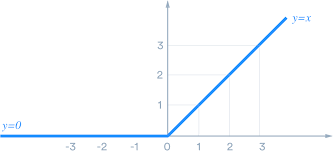Learn to Code via Tutorials on Repl.it

← Back to all posts
Making a neural network with sci-kit learn

# Neural Networks in sci-kit learn.

This tutorial is meant as a follow up to my last neural network tutorial. Today we'll be making a neural network using sci-kit learn.

This tutorial should be slightly shorter as I don't have as much explaining to do because I explained mostly how a neural network works in the last tutorial. The point of the last tutorial was mostly to explain how the neural network works. The point of this one is to provide you with code that can be used in your projects.

One thing that is different from this tutorial and the last is that we will be making a slightly different neural network. We previously made a perceptron which is very simple and has only one hidden layer (a set of nodes which are between the input nodes and the output nodes). This time we are making a multi-layer perceptron, which as you can tell by the name, is basically a perceptron but with more layers.

## Just the code

``````from sklearn.neural_network import MLPClassifier
clf = MLPClassifier(solver='lbfgs', alpha=1e-5,hidden_layer_sizes=(5, 2), random_state=1)
input_train = [1,2,3], [3,2,1]]
output_train = [0, 1]
clf.fit(input_train,output_train)

print((clf.predict([[9,8,6],[6,8,9]])))``````

To use the code simply change the architecture by changing the hidden_layer_sizes in clf and the input_train and output_train arrays to train the neural network accordingly. the predict() function will return an array containing the output values.

## Differences between the MLP and the Perceptron

#### Difference 1: Training

The MLP trains using backpropagation, backpropagation is an algorithm which pretty much finds a way to fine-tune the neural network.
It works by getting the error of a neural network which is pretty much a number showing how far off the neural network was from the true value. It then tries to change the weights of the neural network to get the outputs much closer to the true value.

#### Difference 2: Architecture

The architecture of the perceptron that we built in the last tutorial was three inputs connected to one function node leading to the output. For an MLP, there are various other layers. This can be changed by changing the hidden layers in the code. Currently the architecture is (5,2).

The activation function used in this neural network is RelU. RelU looks like this when plotted on a graph:The activation function we used when making the perceptron was the Sigmoid activation but there are various others such as softmax and tanh.